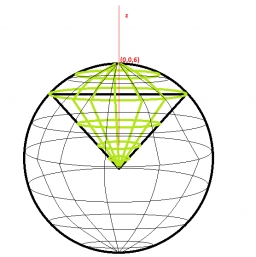# Surface of the cone

Calculate the surface of the cone if its height is 8 cm and the volume is 301.44 cm3.

Correct result:

S =  301.4706 cm2

#### Solution:We would be pleased if you find an error in the word problem, spelling mistakes, or inaccuracies and send it to us. Thank you!Tips to related online calculators
Pythagorean theorem is the base for the right triangle calculator.
Tip: Our volume units converter will help you with the conversion of volume units.

#### You need to know the following knowledge to solve this word math problem:

We encourage you to watch this tutorial video on this math problem:

## Next similar math problems:

• Cone A2VSurface of cone in the plane is a circular arc with central angle of 126° and area 415 cm2. Calculate the volume of a cone.
• Volume of the coneCalculate the volume of the cone if the content of its base is 78.5 cm2 and the content of the shell is 219.8 cm2.In a regular quadrilateral pyramid, the height is 6.5 cm and the angle between the base and the side wall is 42°. Calculate the surface area and volume of the body. Round calculations to 1 decimal place.
• Lateral surface areaThe ratio of the area of the base of the rotary cone to its lateral surface area is 3: 5. Calculate the surface and volume of the cone, if its height v = 4 cm.
• Iglu - cone tentThe cone-shaped tent is 3 m high, the diameter of its base is 3.2 m. a) The tent is made of two layers of material. How many m2 of fabric is needed for production (including flooring), if 20% needs to be added to the minimum amount due to cutting waste? b
• Triangular prism - regularThe regular triangular prism is 7 cm high. Its base is an equilateral triangle whose height is 3 cm. Calculate the surface and volume of this prism.
• Cone containerRotary cone-shaped container has a volume 1000 cubic cm and a height 12 cm. Calculate how much metal we need for making this package.
• Cone sideCalculate the volume and area of the cone whose height is 10 cm and the axial section of the cone has an angle of 30 degrees between height and the cone side.
• Axial section of the coneThe axial section of the cone is an isosceles triangle in which the ratio of cone diameter to cone side is 2: 3. Calculate its volume if you know its area is 314 cm square.
• Triangular prismThe base of the perpendicular triangular prism is a rectangular triangle with a hypotenuse of 10 cm and one leg of 8 cm. The prism height is 75% of the perimeter of the base. Calculate the volume and surface of the prism.
• The diagram 2The diagram shows a cone with slant height 10.5cm. If the curved surface area of the cone is 115.5 cm2. Calculate correct to 3 significant figures: *Base Radius *Height *Volume of the cone
• Sphere parts, segmentA sphere with a diameter of 20.6 cm, the cut is a circle with a diameter of 16.2 cm. .What are the volume of the segment and the surface of the segment?
• 9-gon pyramidCalculate the volume and the surface of a nine-sided pyramid, the base of which can be inscribed with a circle with radius ρ = 7.2 cm and whose side edge s = 10.9 cm.
• CalculateCalculate the surface and volume of the cone that results from the rotation of the right triangle ABC with the squares 6 cm and 9 cm long around the shorter squeegee.
• Four sided prismCalculate the volume and surface area of a regular quadrangular prism whose height is 28.6cm and the body diagonal forms a 50 degree angle with the base plane.
• Cone and the ratioRotational cone has a height 23 cm and the ratio of the base surface to lateral surface is 7: 9. Calculate a surface of the base and the lateral surface.
• The tentThe tent shape of a regular quadrilateral pyramid has a base edge length a = 2 m and a height v = 1.8 m. How many m2 of cloth we need to make the tent if we have to add 7% of the seams? How many m3 of air will be in the tent?